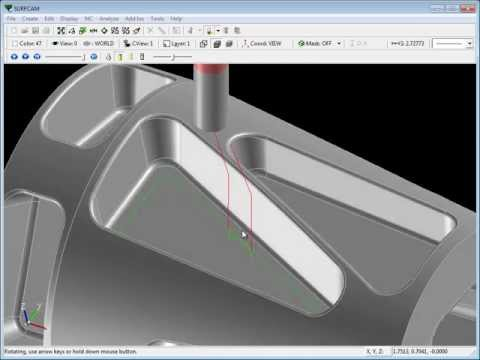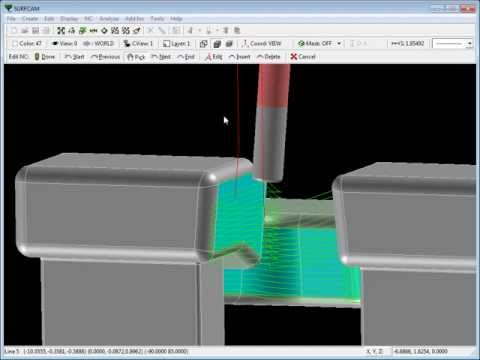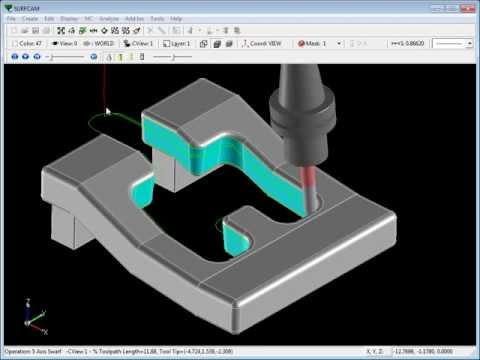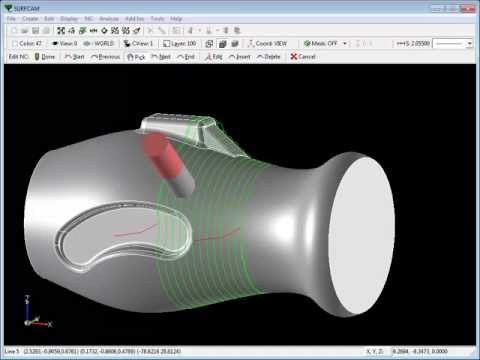SURFCAM Tutorial - Introduction to 5-Axis SwarfSURFCAM Tutorial - Introduction to 5-Axis SwarfSURFCAM Tutorial - Introduction to 5-Axis Swarf, Part 2SURFCAM Tutorial - 5-Axis Swarf: Morph Between Two Curves